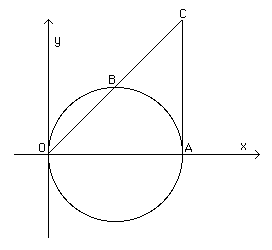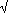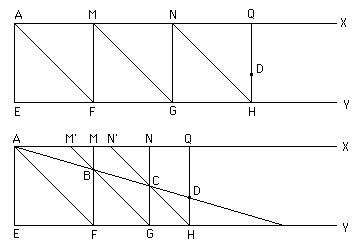# Doubling the cube

There are three classical problems in Greek mathematics which were extremely important in the development of geometry. These problems were those of squaring the circle, doubling the cube and trisecting an angle. Although these are closely linked, we choose to examine them in separate articles. The present article studies the problem of doubling the cube, or duplicating the cube, or the Delian problem which are three different names given to the same classical problem. It is fair to say that although the problem of squaring the circle was to become the most famous in more modern times, certainly among amateur mathematicians, the problem of doubling the cube was certainly the more famous in the time of the ancient Greeks.

Eratosthenes, in his work entitled Platonicus relates that, when the god proclaimed to the Delians through the oracle that, in order to get rid of a plague, they should construct an altar double that of the existing one, their craftsmen fell into great perplexity in their efforts to discover how a solid could be made the double of a similar solid; they therefore went to ask Plato about it, and he replied that the oracle meant, not that the god wanted an altar of double the size, but that he wished, in setting them the task, to shame the Greeks for their neglect of mathematics and their contempt of geometry.

Eutocius, in his commentary on Archimedes' On the sphere and cylinder gave a somewhat different version. This purports to be a letter written by Eratosthenes to King Ptolemy and, although the letter is a forgery, the writer does quote some genuine writings of Eratosthenes :-

The first major step in the problem of doubling the cube was taken by Hippocrates, probably not long after the problem first appeared. However, it seems likely that the problem was already being considered in a more general form, namely:

(i) To find a cube whose ratio to a given cube equals the ratio of two given lines.

Now Hippocrates reduced the problem to the following:

(ii) Given two lines, find two mean proportionals between them.

i.e. given lines a, b find x, y such that a : x = x : y = y : b.

Now it is easy with our modern understanding of ratio to see that (i) and (ii) are equivalent.
For a3 : x3 = (a:x)3 = (a : x)(x : y)(y : b) = a : b.
Thus if we are given a cube with side a and want to construct a cube b : a times the volume, we need to construct the cube of side x.

Now often in articles on doubling the cube the argument of the last paragraph to prove the result of Hippocrates that (i) and (ii) are equivalent is given; see for example . But as pointed out in  this type of argument was not available to Hippocrates so one has to consider not only how he proved the equivalence but also how Hippocrates thought of the result in the first place. There is no way of knowing for certain the answers to these questions. However there are clues which come from the problem of the two dimensional case. Euclid in the Elements, shows that the following two problems are equivalent:

(iii) To find a square whose ratio to a given square equals the ratio of two given lines.

(iv) Given two lines, find one mean proportional between them, i.e. given lines a, b find x such that a : x = x : b.

Again a modern argument gives a2 : x2 = (a : x)2 = (a : x)(x : b) = a : b showing that given a square of side a then, if we construct a square of side x, it has area equal to b : a times that of the square of side a. Euclid, in the Elements Book VI, not only shows the equivalence of (iii) and (iv) but he shows how (iv) can be used to solve (iii). Heath also suggests in  that Hippocrates may have come to the idea from number theory for he quotes Euclid's Elements Book VIII:-

Between two cube numbers there are two mean proportional numbers, and the cube has to the cube the ratio triplicate of that which the side has to the side.

However, a skilful textual analysis of Archimedes' On sphere and cylinder leads the author of  to deduce that compound ratios , while well known to Archimedes, belonged to more modern mathematics than that available to Hippocrates. Whatever the reasoning that took Hippocrates to show that the problem of doubling the cube reduced to (ii), it is quite remarkable that all later mathematicians attacked the problem (ii) rather than the original formulation.

We next consider the solution proposed by Archytas. This is a quite beautiful solution showing quite outstanding innovation by Archytas. Heath writes :-

The solution by Archytas is the most remarkable of all, especially when his date is considered (first half of the fourth century BC), because it is not a plane construction but a bold construction in three dimensions, determining a certain point as the intersection of three surfaces of revolution...

We shall indicate the construction used by Archytas as given by Eutocius. We shall attempt to give a modern interpretation of this construction to make it more understandable but emphasise that the coordinate style in parts of the description are in no way present in Archytas.Consider a circle in the xy-plane with diameter OA on the x-axis, where O is the origin and A the point (a, 0). Let B be the point on the circle with OB = b. The aim is to find two mean proportionals between a and b. Extend OB to meet the tangent to the circle at A at the point C. Suppose we consider this figure as lying in 3-dimensional space where the z-axis is at O coming straight out of the plane of the diagram. Now we imagine the three surfaces of revolution referred to by Heath in the quotation above. One surface is that of a half-cylinder on the circle OBA as base coming out of the plane of the diagram. The second is the surface of the cone produced by OC when the triangle OCA is rotated about the line OA. The third surface is produced by considering a semicircle in the xz-plane with OA as its diameter sitting above the xy-plane and then rotating this semicircle on OA where OA rotates in the xy-plane about O. This surface is one half of a torus with the hole in the centre of the torus being only the point O.

Suppose the three surfaces of revolution intersect at the point P. Then P, being on the half-cylinder lies above a point N on the circle OBA. The two mean proportionals constructed by Archytas are then OP and ON. We shall use some coordinate geometry in a moment to see that Archytas is correct, but first let us give the construction in the words of Eutocius, unchanged except for the names of the point which I have changed to fit the notation of our diagram and that described above (see for example ):-

This is the solution of Archytas, reported by Eudemus:

Let the two given lines be OA [= a] and b; it is required to construct two mean proportionals between a and b. Draw the circle OBA having OA as diameter where OA is the greater [OA = a b]; and inscribe OB, of length b and produce it to meet at C the tangent to the circle at A. ... imagine a half-cylinder which rises perpendicularly on the semicircle OBA, and that on OA is raised a perpendicular semicircle standing on the [base] of the half-cylinder. When this semicircle is moved from A to B, the extremity O of the diameter remaining fixed, it will cut the cylindrical surface in making its movement and will trace on it a certain bold curve. Then, if OA remains fixed, and if the triangle OCA pivots about OA with a movement opposite to that of the semicircle, it will produce a conical surface by means of the line OC which, in the course of its movement, will meet the curve drawn on the cylinder at a particular point [P]. ...

(2) x2 + y2 + z2 = a(x2 + y2)

and the conical surface has equation

(3) x2 + y2 + z2 = a2x2/b2.

If (p, q, r) is the point where these three surfaces intersect then
OP =(p2+q2+r2) while ON =(p2+q2).

Now from (1) and (3) p2+q2+r2 = (p2+q2)2/b2.

Thus

a/(p2+q2+r2) =(p2+q2+r2)/(p2+q2) =(p2+q2)/b

as required.

Through the writings of Eutocius, we know that Eudoxus also gave a solution to the problem of doubling the cube. His solution is lost, however, since the version which Eutocius had in front of him was rather trivially incorrect and he therefore did not reproduce it. Nobody believes that Eudoxus had an elementary error in his solution (he was far too good a mathematician for that) so the error must have been an error introduced when his solution was copied by someone who did not understand it properly. Paul Tannery suggested that Eudoxus's solution was a two-dimensional version of the one given by Archytas which we have just described, in effect the solution obtained by projecting Archytas's construction onto a plane. However, Heath  suggests that Eudoxus was:-

... too original a mathematician to content himself with a mere adaptation of Archytas's method of solution.

I [EFR] agree with this assessment of Heath, so there seems little chance now that we will ever know how Eudoxus solved the doubling of the cube.

Menaechmus is said to have made his discovery of conic sections while he was attempting to solve the problem of doubling the cube. Menaechmus's solution to finding two mean proportionals is described by Eutocius in his commentary to Archimedes' On the sphere and cylinder.

Suppose that we are given a, b and we want to find two mean proportionals x, y between them, i.e. a : x = x : y = y : b. Using modern mathematics, which of course was in no way available to Menaechmus, we can see how the conic sections arise in solving the problem. Now

a/x = y/b so xy = ab,

x/y = y/b so y2 = bx, and

a/x = x/y so x2 = ay.

Menaechmus gave two solutions. The first comes from the rectangular hyperbola and the parabola which are the first two equations in our list. We now see that the values of x and y are found from the intersection of the parabola y2 = bx and the rectangular hyperbola xy = ab. Of course we must emphasis again that this in no way indicates the way that Menaechmus solved the problem but it does show in modern terms how the parabola and hyperbola enter into the solution to the problem. For his second solution Menaechmus uses the intersection of the two parabolas y2 = bx and x2 = ay which are the second and third equations in our list.

One of the great puzzles concerning the solution of the problem of doubling the cube is that there is a mechanical solution known as Plato's machine. Now it seems highly unlikely that Plato would give a mechanical solution, particularly given his views on such solutions. Plutarch wrote (see for example ):-

Plato reproached the disciples of Eudoxus, Archytas and Menaechmus for resorting to mechanics and instrumental means for resolving the problem of duplication of volume; for in their desire to find in some fashion, two mean proportionals, they resorted to a method that was irrational. In proceeding in this way, did not one lose irredeemably the best of geometry, by a regression to a level of the senses, which prevents one from creating and even perceiving the eternal and incorporeal images among which God is eternally god.

There are two theories concerning Plato's machine to solve the doubling of the cube problem. One theory is that Plato invented the mechanical solution to show how easy it is to devise such solutions, but the more widely held theory is that Plato's machine was invented by one of his followers at the Academy.

Eratosthenes is important in the story both because the history of the problem has been communicated through him and also for his own contribution to the problem. He erected a column at Alexandria dedicated to King Ptolemy with an epigram inscribed on it relating to his own mechanical solution to the problem of doubling the cube :-

If, good friend, thou mindest to obtain from any small cube a cube the double of it, and duly to change any solid figure into another, this is in thy power; thou canst find the measure of a fold, a pit, or the broad basin of a hollow well, by this method, that is, if thou thus catch between two rulers two means with their extreme ends converging. Do not thou seek to do the difficult business of Archytas's cylinders, or to cut the cone in the triads of Menaechmus, or to compass such a curved form of lines as is described by the god-fearing Eudoxus. Nay thou couldst, on these tablets, easily find a myriad of means, beginning from a small base. Happy art thou, Ptolemy, in that, as a father the equal of his son in youthful vigour, thou hast thyself given him all that is dear to muses and Kings, and may be in the future, O Zeus, god of heaven, also receive the sceptre at thy hands. Thus may it be, and let any one who sees this offering say "This is the gift of Eratosthenes of Cyrene".So what was the machine which Eratosthenes invented to solve the problem? It consists to two parallel lines with triangles between them as shown in the top diagram. Here AE and DH are the two lengths for which it is required to find two mean proportionals. Now keep the first triangle AMF fixed, but allow the triangles MNG and NQH to slide within the frame bounded by AX and EY. Rotate AX until it passes through D but while doing this make sure that the points B and C where this rotating line cuts MF and NG continue to also lie on the sides MG and NH of the two triangles which move to the left to allow this configuration to remain possible. The triangles slide left until the bottom of the two diagrams is reached. In this final diagram BF and CG are the two mean proportionals between AE and DH.

Now Eratosthenes comments in the quote above that his machine is capable of finding more than two mean proportionals. If one were to require 'a myriad of mean' proportionals, then one would need to put that number of movable triangles into his machine and the same procedure will find the 'myriad' of mean proportionals.

Other solutions to the problem were by Philon and Heron who both gave similar methods. Their solution is effectively produced by the intersection of a circle and a rectangular hyperbola. Nicomedes, who was highly critical of Eratosthenes mechanical solution, gave a construction which used the conchoid curve which he also used to solve the problem of trisection of an angle. Details of the construction is given in . Diocles also invented a special curve to solve the problem of doubling the cube, namely the cissoid curve.

The proof of the impossibility had to await the mathematics of the 19th century. The final pieces of the argument were put together by Pierre Wantzel. In 1837 Wantzel published proofs in Liouville's Journal of:-

Gauss had stated that the problems of doubling a cube and trisecting an angle could not be solved with ruler and compasses but he gave no proofs. In this 1837 paper Wantzel was the first to prove these results. Improved proofs were later given by Charles Sturm but he did not publish them.

Article by: J J O'Connor and E F Robertson

References (8 books/articles)

JOC/EFR April 1999